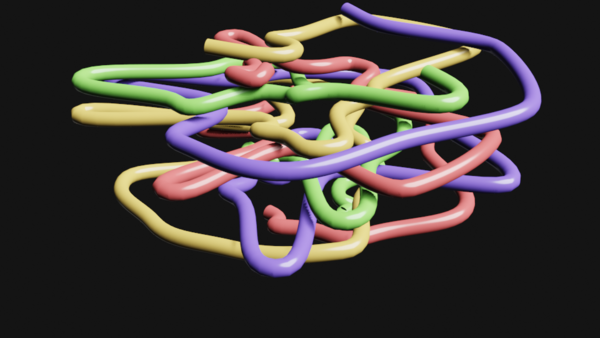## blender 2.80 experiment 5 - scripted curve

For this blender 2.80 experiment I created four bezier curves using the blender python api. There have been some changes in the api, but porting existing python scripts to 2.80 is pretty easy - the main changes required for older code to run is how the objects get added to the scenes, because of the new collections in blender 2.80to run a python script open a texteditor and run a script with ALT-p

the script I used to create the curves is

``````import bpy
from math import pi,cos,sin
import random

for curves in range(4):
cd = bpy.data.curves.new(name='Curve%d' %(curves), type='CURVE')
cd.dimensions='3D'

b = cd.splines.new('BEZIER')

for i in range(60):
a =  i/10.0 * 2.0*pi + pi/2.0*curves
r = 0.5
xh1 = cos(a + pi/2.0) * r
yh1 = sin(a + pi/2.0) * r
xh2 = cos(a - pi/2.0) * r
yh2 = sin(a - pi/2.0) * r

r2 = random.uniform(0.1,3)

x = cos(a)*r2
y = sin(a)*r2
if i > 0:
b.bezier_points[-1].handle_right = (x+xh1,y+yh1,i/10)
b.bezier_points[-1].handle_left = (x+xh2,y+yh2,i/10)
b.bezier_points[-1].co = (x,y,i/10)

od = bpy.data.objects.new('CurveObject%d'%(curves), cd)
od.location=(0,0,0)
od.data.resolution_u=12
od.data.bevel_resolution=12
od.data.fill_mode = 'FULL'
od.data.bevel_depth=0.1